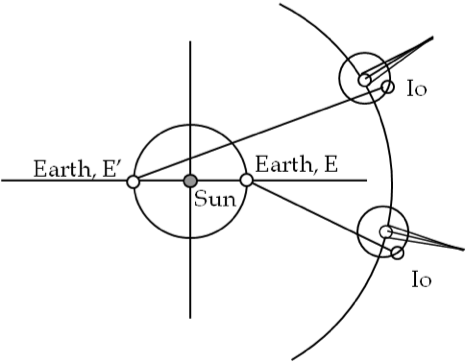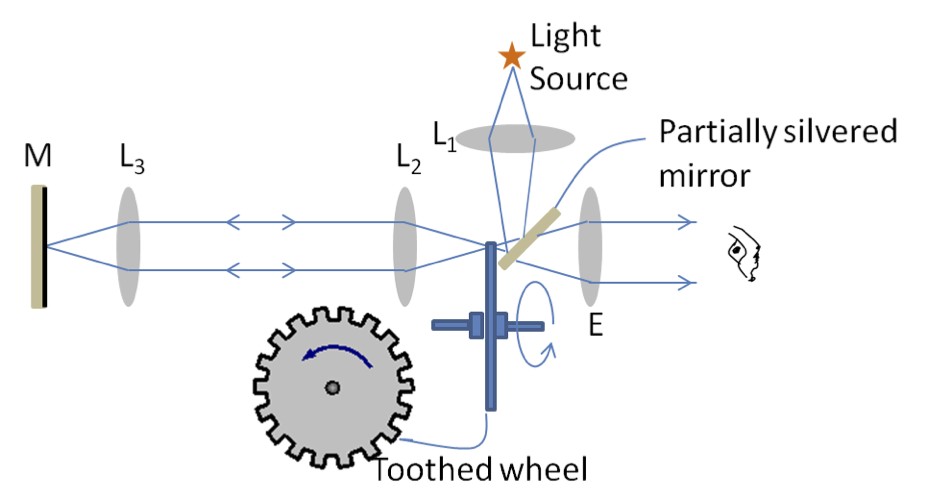## Section46.3Speed of Light

Light in vacuum is the fastest speed known to mankind. In most everyday situation, it appears to go from one place to another in “no time”. But, over the years we have learned to measure speed of light more accurately.

Galileo's Unsuccessful Attempt:

Perhaps the first attempt at measuring the speed of light was made by Galileo Galilei of Italy in early 1600s. Galileo and an assistant each carried a lantern with a shutter and placed themselves on different hilltops about a mile apart. As Galileo opened the shutter, his assistant was to open his shutter as soon as he saw the light from Galileo's lantern. Galileo will then record the time for the round trip. He found no difference in the result from the result on the same experiment conducted with the lanterns only a few meters apart.

From his experiments Galileo could not draw any conclusion about the speed of light because the speed of light is too great to be measured by his method. As a matter of fact, it takes only about $11\ \mu\text{s}$ for light to travel the round trip between two hilltops separated by 1 mile. Since the reaction time for Galileo and his assistant was of the order of hundreds of milliseconds, there was no way of determining the speed of light with the time measuring technique utilized by Galileo.

Römer's Ingenous Idea:

Of course if the distance for the travel of light in Galileo's experiment were large, then we may be able to determine the speed of light by his method. Astronomical objects do provide large distances and could be used to deduce speed of light if we know the distance by some other means. In 1675 the Danish astronomer Ole Römer noticed something strange in the motion of the largest and brightest moon Io about planet Jupiter. The basic observations of Römer are illustrated in Figure 46.3.1. From his observations of the moon Io he deduced that the time required for the light to travel a distance equal to the diameter of the orbit of the Earth about the Sun is equal to 16 minutes and 36 seconds.Figure 46.3.1. Illustrating Roemer's determination of speed of light based on observations made on Jupiter's moon Io.

The moon Io goes behind the Jupiter once every time Io goes around the planet. The eclipse of Io by Jupiter is easily observed fro Earth with good accuracy. The interval between the successive eclipses of Io gives a measure of its orbital time period, which was of considerable interest at the time - it was to serve as a clock in the sky for accurate navigation purposes.

Römer measured the timing of eclipses of Io and notices that the interval betwen successive eclipses of Io, which occur every 1.769 Earth days, became shorter as distances between Earth and Jupiter became less and became longer as distances between Earth and Jupiter became more dure to Earth's orbit around Earth.

From his data, Römer estimated that timing between successive eclipses of Io by Jupiter when Earth and Jupiter were closest (Earth at E) and when Earth and Jupiter were furthert (Earth at E$^{\prime}$), shown in Figure 46.3.1, was 22 minutes (actually, the correct value as known now is 16.7 minutes). Römer correctly interpreted this discrepancy to be the difference in successive times to be indicative of the longr distance light has to travel from Io to Earth when earth is at E$^{\prime}$ versus it is at E.

But Römer didn't use his insight to calclate speed of light. Instead, a contemporary Dutch physicist Christian Huygens used Römer's observed time delay and approximately 280 million km for whole diameter (EE$^{\prime}$) of earth's orbit to estimate the speed of light for the first time.

\begin{equation*} c \approx = \frac{280\times 10^9\text{ m}}{22\times 60\text{ s}} = 2.12\times 10 ^{8}\ \text{m/s}. \end{equation*}

This estimate of speed of light is about $30\%$ lower than the exact value determined by other techniques.

Fizeau's Experiment:

The first successful direct measurement of the speed of light was made by Armand Hippolyte Louis Fizeau (1819-1896) in 1849. Fizeau's experiment was similar in spirit to that of Galileo in that he measured time for a roundtrip for light. To be able to measure small time interval involved, Fizeau came up with an ingenious arrangement shown in Figure 46.3.2.

In Fizeau's experiment, light passed through a rotating cogwheel with toothed edges before being reflected off a mirror placed approximately five and half miles away. If the wheel was not rotating at the right speed, the returning light will strike a tooth in the wheel and will be blocked. But, if the cogwheel rotated at just the right speed, light would go out from one opening in the cogwheel and return though the next opening.Figure 46.3.2. Schematic drawing of Fizeau's apparatus. The light from a source is focused on a toothed wheel by lens $L_1$ which is at the focal point of lens $L_2$ which makes the light paralell to the axis. The light then travels to the mirror M and reflected back. If the tooth is not blocking the ray, then the ray passes to the eyepiece E and to the eye.

Let there be N teeth in the wheel. Let the total distance traveled by the light be $2d\text{.}$ Suppose the light is leaving one opening in the toothed wheel makes it through the very next opening when the wheel rotates at the rate $n$ revolutions per second. You can show that this will give the speed of light to be $2nNd\text{.}$ In Fizeau's experiment values were $d = 8.63\text{ km}\text{,}$ $N = 720\text{,}$ $n = 25 \text{ revs per sec}\text{.}$ This gave the following value of speed of light.

\begin{equation*} v = 2nNd = 3.13 \times 10^8\text{ m/s}. \end{equation*}

This is just $4.3\%$ from the exact value.

Foucault's Experiment:

Jean Bernard Leon Foucault (1819-1868) improved on the apparatus of Fizeau by replacing the rotating cogwheel with a rotating plane mirror. A simple version of Foucault's experiment is shown in Figure 46.3.3. Light from a source S is reflected from the rotating mirror M towards a concave mirror N. The reflected ray from N travels along the original path to M. If the mirror M is not rotating, then the return ray forms an image of the source at I. However, when the mirror M is rotating, then the reflected ray from the concave mirror finds the mirror M in a new orientation $\text{M}^{\prime}\text{.}$ The reflected ray from the new position of the plane mirror forms the image at $I^{\prime}\text{.}$

Foucault found that the mirror N with a radius of curvature of $R = 20\text{ m}$ and a rate of rotation of $800\text{ rev per sec}$ for the mirror M, the image was displaced by $x = 0.7\text{ mm}\text{.}$ This gave the speed of light

\begin{equation*} v = 298,000\text{ km/s}. \end{equation*}

This is only $0.6\%$ lower than the currently accepted value of $299,792,458\text{ m/s}\text{.}$

Michelson's Experiment:

In 1920's, Albert Michelson in the United States conducted experiments measuring the speed of light using a rotating prism as shown in Figure 46.3.4. Michelson set his apparatus at Mount Wilson Observatory in Southern California and the reflecting mirror on the Lookout Mountain of Mount San Antonio, about $22\text{ miles}$ away. Careful measurements of Michelson led to a more precise value of the speed of light to be

\begin{equation*} v = 299,796\pm4\text{ km/s}. \end{equation*}

### Subsection46.3.1SI Unit of Length

The measured speed of light in vacuum in the experiments mentioned above uses a standard meter for the distance traveled by light. The 1983 Conference Generale des Poids et Mesures turned the table around, and adopted an exact value of speed of light to be $299,792,458\text{ m/s}\text{,}$ and defined the unit of meter as the length of the path traveled by light in vacuum during a time interval of $1/299,792,458$ of a second.

\begin{equation} c = 299,792,458\ \text{m/s}\ \ \ \text{(Exact)}\tag{46.3.1} \end{equation}

With an exact value assigned to the speed of light, the unit of length, viz. $\text{meter, m}\text{,}$ depends on the choice of the unit of time. Currently, the unit of time, viz., $\text{second, s}\text{,}$ is defined in terms of frequency of light emitted by excitation of krypton atoms.

The average distance between the Earth and the Sun is $1.5\times10^8\text{ km}\text{.}$ Find the time light takes to get from the Sun to the Earth.

Hint

Use $d=ct\text{.}$

$500\text{ s}\text{.}$

Solution

We use $d = ct$ to find time $t\text{.}$

\begin{align*} t \amp = \dfrac{d}{c} = \dfrac{1.5\times 10^{8}\:\text{km}}{3\times 10^{8}\:\text{m/s}} \\ \amp = 500\:\text{s} = 8\:\text{min and }\: 20\:\text{s}. \end{align*}

The nearest star is approximately 4.2 light-years away from the Earth. How far is the distance in kilometers? A lightyear is a distance light travels in vacuum in one Earth Julian year, which is 365.25 days.

Hint

Multiple time and speed.

$9.46\times 10^{12}\text{ km}\text{.}$

Solution

Multiplying time in one year by speed $c= 299792.458\text{ km/s}$ we get one lightyear to be the following distance in $\text{km}\text{.}$

\begin{align*} d \amp = c t\\ \amp = 299792.458 \times 365.25\times 24\times 3600= 9.46\times 10^{12}\text{ km}. \end{align*}

Slits at the circumference of a rotating circular disk of radius $3\text{ cm}$ are made so that the distance between the slits is $0.1\text{ mm}\text{.}$ A ray of light passes through one slit and is reflected off a mirror at a distance of $50\text{ m}$ from the disk. Upon return the ray passes through the adjacent slit in place due to ratation. Find the rotation speed of the wheel.

Hint

Equate the time for slit to move one slit separation and the time for light to go to mirror and return.

$1590\text{ rev/sec}\text{.}$

Solution

The following figure shows the layout of the experiment.

We will equate the time for slit to move one slit separation and the time for light to go to mirror and return. This is the necessary condition.

The velocity at the rim will be $v = \omega R\text{.}$ Therefore, the time $\Delta t$ to cover a distance $s$ at the rim will be

\begin{equation*} \Delta t = \dfrac{s}{v} = \dfrac{s}{\omega R} = \dfrac{0.0001\:\text{m}}{0.03\:\text{m}\times \omega} = \dfrac{0.01}{3}\:\dfrac{1}{\omega}. \end{equation*}

During this time light travels $50 + 50 = 100$ m. Therefore,

\begin{equation*} \dfrac{0.01}{3}\:\dfrac{1}{\omega} = \dfrac{100\:\text{m}}{ 3\times 10^{8}\:\text{m/s}}. \end{equation*}

Solving for $\omega$ we find

\begin{equation*} \omega = \dfrac{\frac{1}{3}\times 0.01}{\frac{1}{3}\times 10^{-6}} = 10^{4}\:\text{rad/s}. \end{equation*}

This can be written as revolutions per sec if we divide this result by $2\pi$ rad in one revolution.

\begin{equation*} \omega = 10^{4}\:\text{rad/s} = \frac{10^{4}}{2\pi}\:\text{rev/s} \approx 1590 \:\text{rev/s}. \end{equation*}